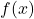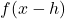## Identify the effect on the graph of replacing f(x) by A f(x)

Question

Identify the effect on the graph of replacing f(x) by A f(x)

in progress 0
2 months 2021-07-24T15:09:00+00:00 1 Answers 2 views 0

See explanation

Step-by-step explanation:

Required

Effect of replacingwithf(x) is represented as: (x,y)

While

f(x – h) is represented as (x – h, y)

Notice the difference in both is that, the x value in f(x – h) is reduced by a constant h while the y value remain unchanged.

This means that the graph of f(x) will shift horizontally (i.e. along the x-axis) to the left by h units